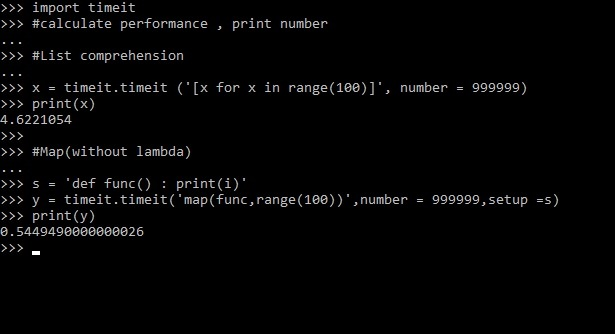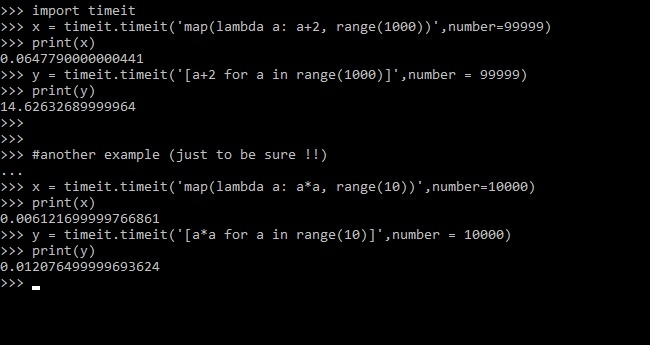# Python – Map vs List comprehension

• Difficulty Level : Expert
• Last Updated : 30 Jan, 2020

Suppose we have a function and we want to compute this function for different values in a single line of code . This is where map() function plays its role ! `map()` function returns a map object(which is an iterator) of the results after applying the given function to each item of a given iterable (list, tuple etc.)

Syntax: map(funcname, iterables)

Attention geek! Strengthen your foundations with the Python Programming Foundation Course and learn the basics.

To begin with, your interview preparations Enhance your Data Structures concepts with the Python DS Course. And to begin with your Machine Learning Journey, join the Machine Learning - Basic Level Course

Parameters:

funcname: It is the name of the function which is already defined and is to be executed for each item.
iterables: It can be list, tuples or any other iterable object.

Return Type: Returns a map object after applying the given function to each item of a given iterable (list, tuple etc.)

Example:

 `# function to double the number``def` `num (n) :``    ``return` `n ``*` `2``         ` `lst ``=` `[``2``, ``44``, ``5.5``, ``6``, ``-``7``]`` ` `# suppose we want to call function``# 'num' for each element of lst,``# we use map`` ` `# creates a map object``x ``=` `map``(num, lst) ``print``(x) `` ` `# returns list``print``(``list``(x))  `

Output:

```<map object at 0x7f859f3f05c0>
[4, 88, 11.0, 12, -14]
```

List Comprehension is a substitute for the lambda function, `map()`, `filter()` and `reduce()`. It follows the form of the mathematical set-builder notation. It provide a concise way to create lists.

Syntax:

```[ expression for item in list if conditional ]
```

Parameters:

• Expression – based on the variable used for each element
• for ..in – ‘for’ followed by the variable name to use, followed by ‘in’
• if – to filter

Example:

 `lst ``=` `[``2``, ``44``, ``5.5``, ``6``, ``-``7``]`` ` `# to double the number``# list comprehension``x ``=` `[i ``*` `2` `for` `i ``in` `lst ] ``print``(x)`

Output:

```[4, 88, 11.0, 12, -14]
```

#### Map VS List Comprehension

• List comprehension is more concise and easier to read as compared to map.
• List comprehension allows filtering. In map, we have no such facility. For example, to print all even numbers in range of 100, we can write `[n for n in range(100) if n%2 == 0]`. There is no alternate for it in map
• List comprehension are used when a list of results is required as map only returns a map object and does not return any list.
• List comprehension is faster than map when we need to evaluate expressions that are too long or complicated to express
• Map is faster in case of calling an already defined function (as no lambda is required).

#### Comparing Execution Time

Note : We will be using an in-built python library ‘`timeit`‘.

• Without lambda

Let us take a simple operation to print number in a given range. We perform this operation using both map and list comprehension one by one.From the above Example, we can conclude that map performs better than list comprehension when a function is defined already.

• With lambda in map
Let us take operations where we require a lambda inside the map(). The first operation is to add 2 to every number in the given range. Second operation is to square every number in the given range.From the above code, we can observe that map still works better than list comprehension.

Note: There are cases when list comprehension can perform better than map where expressions are too long and complex.

My Personal Notes arrow_drop_up